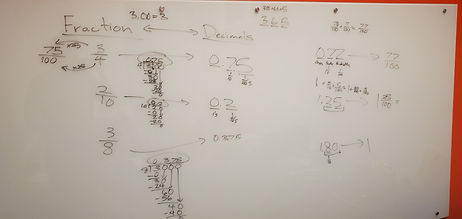Mr. Jake

Target 1​

Lesson Type:

Continuation

Number Operation

:

Fractional Numbers

Convert between fractions, decimals, and percentages.

1:

Convert fractions to decimals and decimals to fractions.

2:

Understand that the digit to the left of the decimal is the whole number, the digits to the right are the numerator, and the denominator is the place-value of the furthest right number.

5th

Vocabulary:

Fraction, Decimal, Convert

Activities:

Practiced converting between fractions and decimals using knowledge of place values

Converted fractions to decimals using divisionHome Exploration

Guiding Questions:Absent Students:

Target 2

:

1:

Understand that a function is a rule that gives one output to each input.

2:

Understand that by studying the proportional relationship between inputs and outputs, a function (equation) can be derived.

3:

Use a function equation to fill out a function table.

7th

Vocabulary:

Function, Rule, Equation, Input, Output, Variable

Activities:

Used a equation to fill out a function table

Created equations that represent functionsHome Exploration

Guiding Questions:Target 3

:

Vocabulary:

Activities:Home Exploration